# How to Sum by Formula if Cell Contains Both A and B in Excel

In previous articles, we have introduced the method of sum numbers for cells which are equal to a certain value or are not equal to a certain value (you can refer to ‘How to Sum if Cell Contains Text in Another Column’, ‘How to Sum Numbers by Formula if Cells Are Not Equal to Certain Value’ on our website). In this article, we will show you the how to ‘sum if cell contains both A and B’ by formula with the help of Excel SUMIFS function.

Through demonstrate a simple instance, we will introduce you the syntax, arguments of SUMIFS function, and let you know how this formula works to reach your goal. After reading the article, you may have a simple understanding of SUMIFS function.

EXAMPLE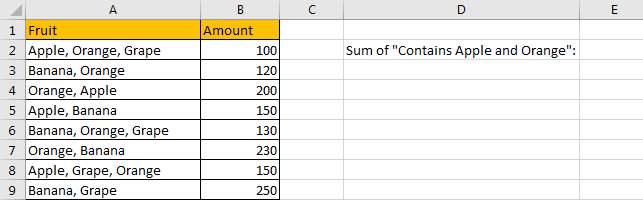Refer to above table, we can see that some kinds of fruit are listed in “Fruit” column. At least two fruits are displayed in one cell. Total amount for fruit combination is listed in “Amount” column accordingly.

In this instance, we want to calculate total amount for fruit combination that contains “Apple” and “Orange”. So, no matter “Apple, Orange” or “Apple, Orange, Grape”, they are both seem to match the criterion. We need to find out all proper fruit combination, and then sum up values in “Amount” column. To fix this issue by formula, we can apply SUMIFS function here.

FORMULA – SUMIF FUNCTION

Step 1: Select A2:A9, then in Name Box define a new name for this range, for example ‘Fruit’.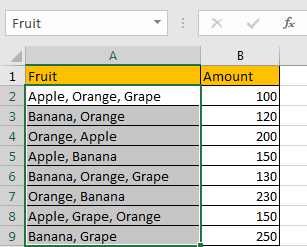Step 2: Select B2:B9, in Name Box define a new name for this range, for example ‘Amount’.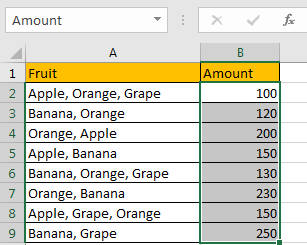Step 3: In E2, enter the formula =SUMIFS(Amount,Fruit,”*Apple*”,Fruit,”*Orange*”).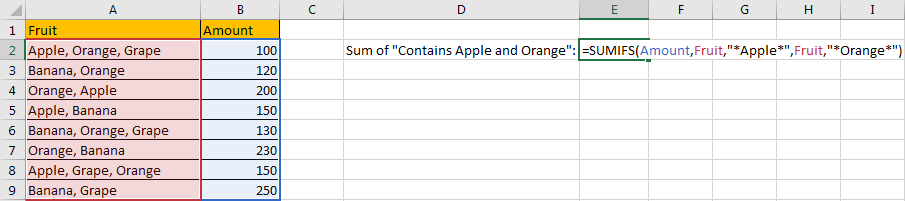Step 4: Press Enter after typing the formula.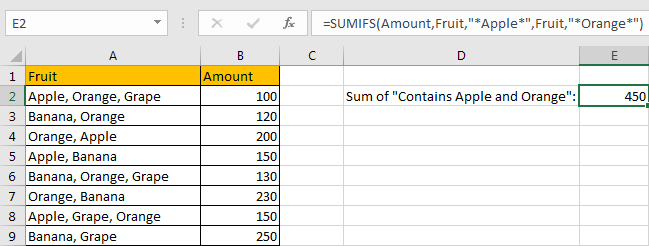We can see in column A, cell A2, A4 and A8 contains both “Apple” and “Orange”, the corresponding amounts are 100 (in cell B2), 200 (in cell B4), and 150 (in cell B8), so the total is 100+200+150=450. The formula works correctly.

SUMIFS FUNCTION INTRODUCTION

SUMIFS function can be seen as SUM+IFS, it can handle multiple ‘criteria range’ and ‘criteria’ combinations.

For SUMIFS function, the syntax is:

SUMIFS(sum_range, criteria_range1, criteria1, [criteria_range2, criteria2], …). Contents in [] are optional.

SUMIFS function supports wildcards like asterisk ‘*’ and question mark ‘?’, it also supports logical operators within its arguments. If wildcards or logical operators are required, they should be enclosed into double quotes (““) with text.

The usage of wildcards:

1. An asterisk (*) means one or more characters.
2. A question mark (?) means one character.
3. The position of asterisk or question mark means the character(s) position relative to the entered part.

The usage of logical operators:

1. “>” – greater than
2. “<” – less than
3. “<>” – not equal to

ALL ARGUMENTS

SUMIFS – SUM RANGE

In our instance, B2:B9 is the ‘sum range’ obviously. Amount values are listed in this field. We define this range with name ‘Amount’ in above step#2.

In the formula bar, select ‘Amount’, press F9, values in this range are listed in an array.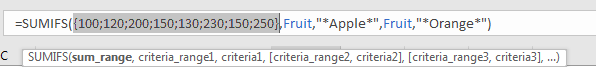SUMIFS – CRITERIA RANGE 1

A2:A9 is the criteria range. In this instance we have only one criteria range. This range contains several kinds of fruit.

In the formula bar, these fruits are displayed in proper order in an array: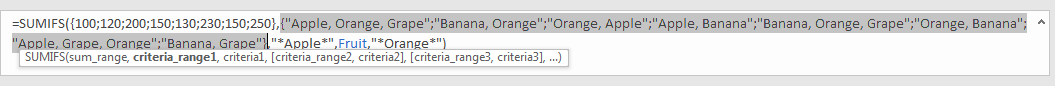SUMIFS – CRITERIA 1

We want to calculate total amount for combinations contain “Apple” and “Orange”, so we will supply two criteria in this case, the first one is “Apple”. As there might be some characters listing before or after “Apple”, so we need to add “*” before and after “Apple”. As SUMIFS function supports texts and wildcards, but they should be enclosed into double quotes “ “ when applying them, so for criteria 1, we enter “*Apple*” in this position.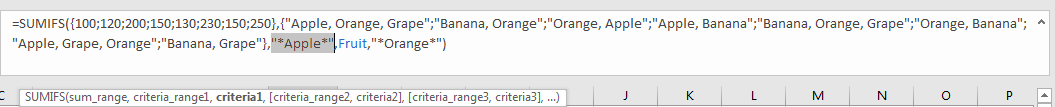SUMIFS – CRITERIA RANGE 2

We have only one criteria range. So in criteria range2 position, we still enter “Amount”.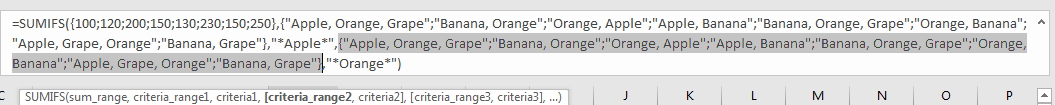SUMIFS – CRITERIA 2

In criteria 1 we enter “*Amount*”, so in criteria 2 we enter “*Orange*”. Then the two criteria are both included in this formula.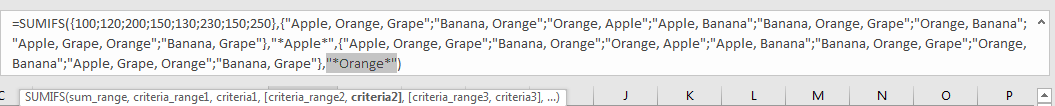HOW FORMULA WORKS

After explaining each argument in the formula, now we will show you how the formula works with these arguments.

Refer to above mentioned arguments, we can get three arrays and two texts within double quotes, the formula is converted into below format in the formula bar.

=SUMIFS({100;120;200;150;130;230;150;250},{“Apple, Orange, Grape”;”Banana, Orange”;”Orange, Apple”;”Apple, Banana”;”Banana, Orange, Grape”;”Orange, Banana”;”Apple, Grape, Orange”;”Banana, Grape”},”*Apple*”,{“Apple, Orange, Grape”;”Banana, Orange”;”Orange, Apple”;”Apple, Banana”;”Banana, Orange, Grape”;”Orange, Banana”;”Apple, Grape, Orange”;”Banana, Grape”},”*Orange*”)

See screenshot below: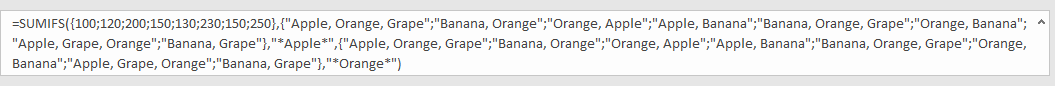There are two pairs of criteria range and criteria:

Criteria Range 1: {“Apple, Orange, Grape”;”Banana, Orange”;”Orange, Apple”;”Apple, Banana”;”Banana, Orange, Grape”;”Orange, Banana”;”Apple, Grape, Orange”;”Banana, Grape”}

Criteria 1: “*Apple*”

Criteria Range 2: {“Apple, Orange, Grape”;”Banana, Orange”;”Orange, Apple”;”Apple, Banana”;”Banana, Orange, Grape”;”Orange, Banana”;”Apple, Grape, Orange”;”Banana, Grape”}

Criteria 2: “*Orange*”

In criteria range 1, compare each fruit combination in the array with criterion “Apple”; if “Apple” exists in fruit combination, mark them in bold:

{“Apple, Orange, Grape“;”Banana, Orange”;”Orange, Apple“;”Apple, Banana“;”Banana, Orange, Grape”;”Orange, Banana”;”Apple, Grape, Orange“;”Banana, Grape”}

So, for the combination which meets the criteria, record a ‘True’ in the array; otherwise, record a ‘False’. Then we can get a new array:

Array 1: {True;False;True;True;False;False;True;False}

In criteria range 2, compare each fruit combination in the array with criterion “Orange”; if “Orange” exists in fruit combination, mark them in bold:

{“Apple, Orange, Grape“;”Banana, Orange“;”Orange, Apple“;”Apple, Banana”;”Banana, Orange, Grape“;”Orange, Banana“;”Apple, Grape, Orange“;”Banana, Grape”}

Then we get another array:

Array 2: {True;True;True;False;True;True;True;False}

As the two criteria “Apple” and “Orange” both should be included in the fruit combination, so we need to look up the intersection of array1 and array2, only True is marked in the same position in two arrays, True will keep in array. So, after comparing the two arrays, we get a new array3:

Array 3: {True;False;True;False;False;False;True;False}

Convert ‘True’ to ‘1’ and ‘False’ to ‘0’:

Array 4: {1;0;1;0;0;0;1;0}

Now, we have below two arrays:

Sum range: {100;120;200;150;130;230;150;250}

Array 4: {1;0;1;0;0;0;1;0} – from above steps we know that if cell contains both “Apple” and “Orange”, 1 is displayed.

We list the two arrays in two rows, and multiply the two numbers in the same column, and save their product in sum total.

Above all, numbers which are not zero in sum total row are the amounts of cell contains both ‘Apple’ and ‘Orange’. Now, sum up all numbers in sum total.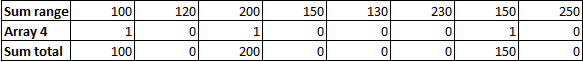100+200+150=450

In this case, if we forgot to add “*” in criteria, let’s see what do we get.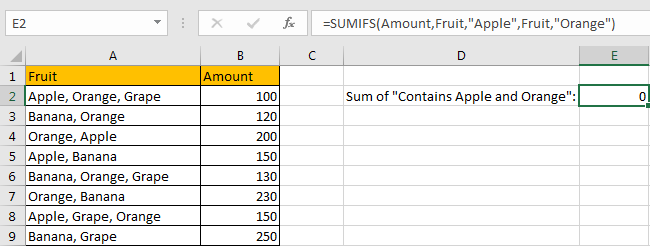In fact, an asterisk (*) means one or more characters, if * is omit in criteria, that means cell which is exactly equal to the criteria can satisfy the condition. As we all know, a cell cannot either be ‘Apple’ or ‘Orange’. So, if we enter =SUMIFS(Amount,Fruit,”Apple”,Fruit,”Orange”) to sum numbers, it doesn’t work.

SUMMARY

1. SUMIFS function can handle multiple groups of criteria ranges and criteria. Sum range is the first argument among all arguments.

2. It supports user defined range name.

3. It supports wildcard.

4. It supports logical operators.

### Related Functions

• Excel SUMIF Function
The Excel SUMIF function sum the numbers in the range of cells that meet a single criteria that you specify. The syntax of the SUMIF function is as below:=SUMIF (range, criteria, [sum_range])…
• Excel SUMIFS Function
The Excel SUMIFS function sum the numbers in the range of cells that meet a single or multiple criteria that you specify. The syntax of the SUMIFS function is as below:=SUMIFS (sum_range, criteria_range1, criteria1, [criteria_range2, criteria2], …)…